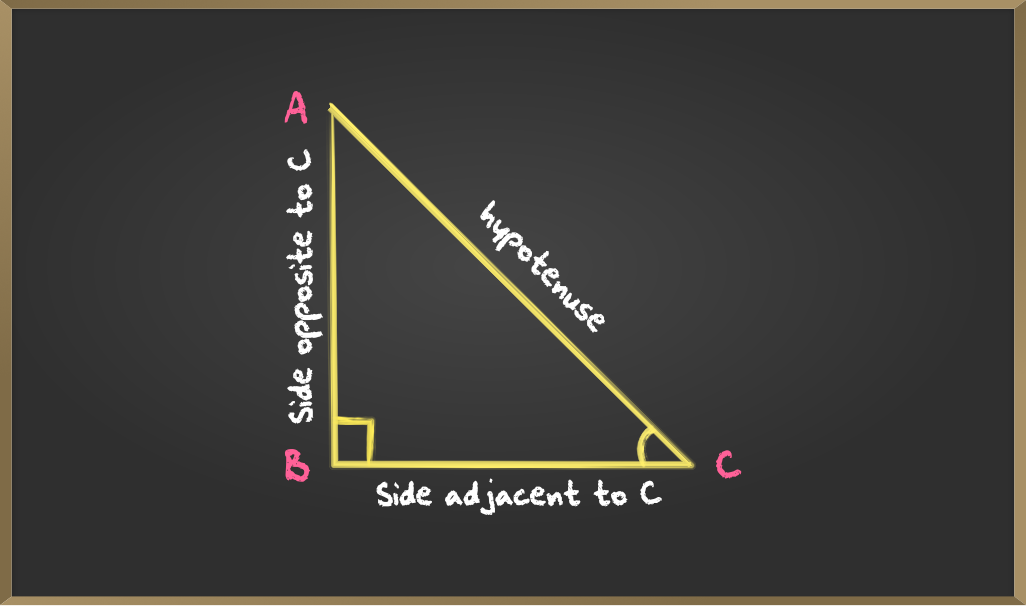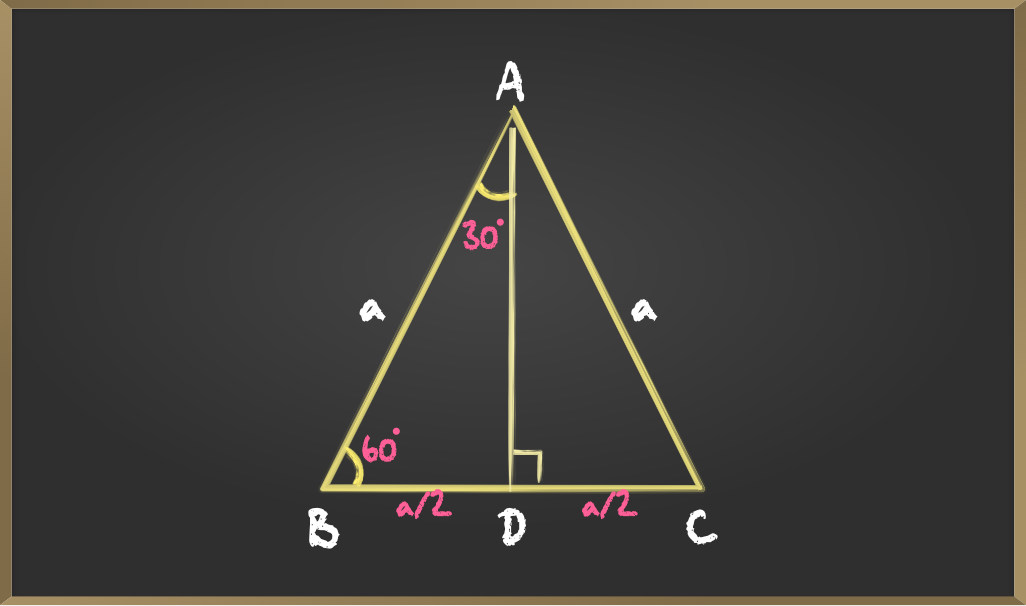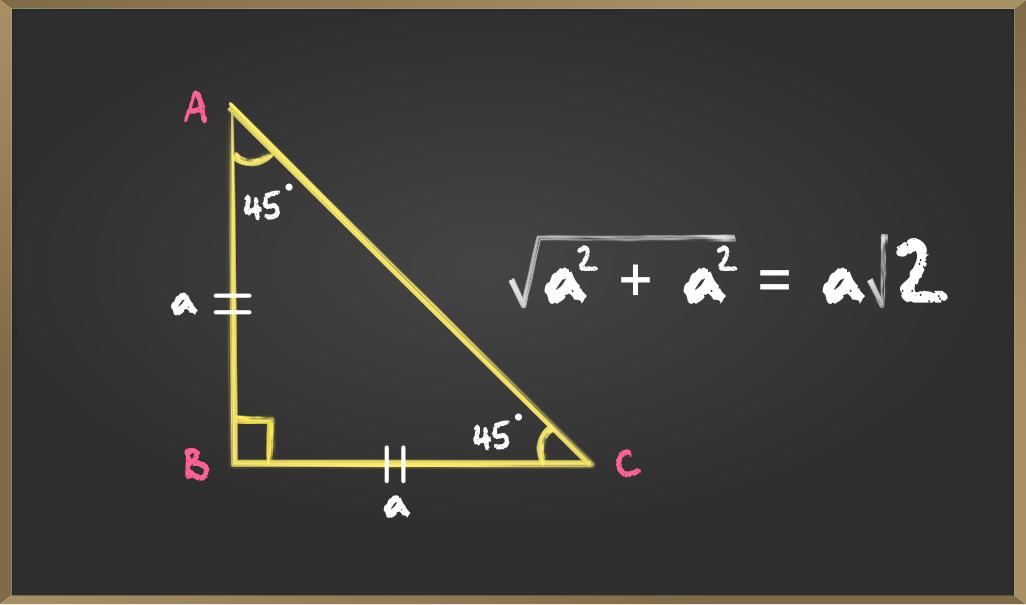Related Articles
Trigonometric ratios of some Specific Angles
• Last Updated : 23 Mar, 2021

Trigonometry is all about triangles or to more precise about the relation between the angles and sides of a right-angled triangle. In this article we will be discussing about the ratio of sides of a right-angled triangle respect to its acute angle called trigonometric ratios of the angle and find the trigonometric ratios of specific angles: 0°, 30°, 45°, 60°, and 90°.

Consider the following triangle:The side BA is opposite to angle ∠BCA so we call BA the opposite side to ∠C and AC is the hypotenuse, the other side BC is the adjacent side to ∠C.

## Trigonometric Ratios of angle C

Sine: Sine of ∠C is the ratio between BA and AC that is the ratio between the side opposite to C and the hypotenuse.Cosine: Cosine of ∠C is the ratio between BC and AC that is the ratio between the side adjacent to C and the hypotenuse.Tangent: Tangent of ∠C is the ratio between BA and BC that is the ratio between the side opposite and adjacent to CCosecant: Cosecant of ∠C is the reciprocal of sin C that is the ratio between the hypotenuse and the side opposite to C.Secant: Secant of ∠C is the reciprocal of cos C that is the ratio between the hypotenuse and the side adjacent to C.Cotangent: Cotangent of ∠C is the reciprocal of tan C that is the ratio between the side adjacent to C and side opposite to C.## Finding trigonometric ratios for angle 0°, 30°, 45°, 60°, 90°

### A. For angles 0° and 90°

If an angle A = 0° then the length of the opposite side would be zero and hypotenuse = adjacent side and if A = 90° then the hypotenuse = opposite side. So by using the above formulas for the trigonometric ratios and if the length of the hypotenuse is a.

if A = 0°if A = 90°Here csc 0, cot 0, tan 90 and sec 90 are not defined as at the particular angle it is divided by 0 which is undefined.

### B. For angles 30° and 60°

Consider an equilateral triangle ABC. Since each angle in an equilateral triangle is 60°, therefore,

∠A = ∠B = ∠C = 60°.∆ABD is a right triangle, right-angled at D with ∠BAD = 30° and ∠ABD = 60°,

Here ∆ADB and ∆ADC are similar as they are Corresponding parts of Congruent triangles(CPCT).Now we know the values of AB, BD, and AD, So the trigonometric ratios for angle 30 areFor angle 60°### C. For angle 45°

In a right-angled triangle if one angle is 45° then the other angle is also 45° thus making it an isosceles right angle triangleIf the length of side BC=a then length of AB=a and Length of AC(hypotenuse) is a√2, then### Result:

My Personal Notes arrow_drop_up